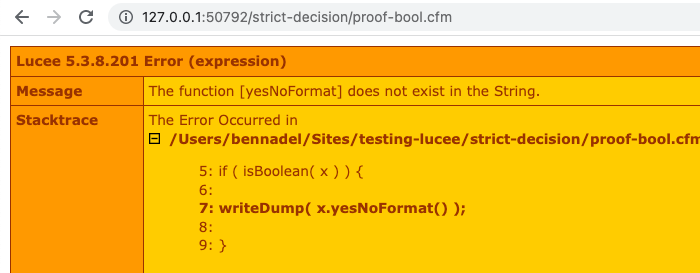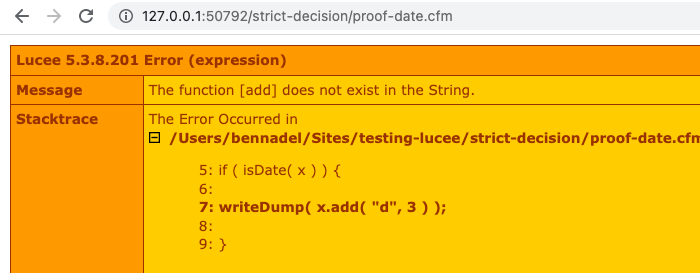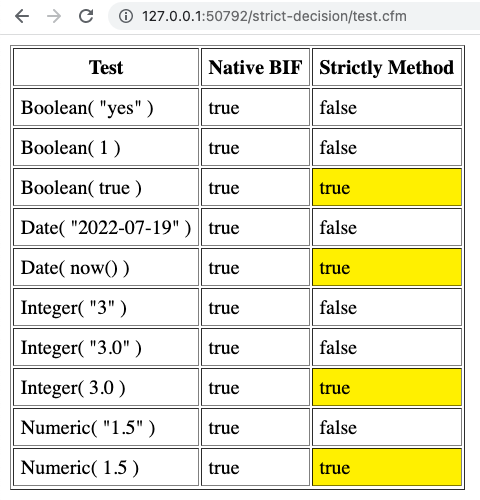# Using Strict Type Decision Methods In ColdFusion

By on
Tags:

Historically, one the nicest features of ColdFusion is the fact that it is loosely typed. And, that the language will - in most cases - happily and transparently cast one data type to another in order to get the job done. As the language has continued to modernize, however, some of its loose behaviors can cause unexpected errors. As such, I was proposing yesterday, on the Lucee CFML developer forums, that the language might introduce some Strict decision functions.

Strict decision functions may not actually make a difference, in terms of a broader issue; but, this is still a fun topic to explore.

Let me illustrate my thoughts here. Imagine the value `"No"`. In any other language, this is a `String`. And, in ColdFusion, it's also a `String`. But, in ColdFusion it's also sometimes a `Boolean` as well. That said, it's not always a `Boolean`; and in those cases, if you try to use it like a `Boolean`, things will go wrong.

First, let's look at an "historic" approach to printing a Boolean value as a "Yes"/"No":

``````<cfscript>

x = "NO";

if ( isBoolean( x ) ) {

writeDump( yesNoFormat( x ) );

}

</cfscript>
``````

Here, we're checking to see if the value is a "Boolean"; and, if so, outputting it using a "Yes"/"No" format. In this case, it doesn't really matter that the value is actually a String because the language knows how to cast it to a Boolean when it needs to.

But, as we modernize the language and add features like member methods, we can start to run into trouble, even if we try to take precautions:

``````<cfscript>

x = "NO";

if ( isBoolean( x ) ) {

writeDump( x.yesNoFormat() );

}

</cfscript>
``````

Here, we're writing the same code; only, instead of using the "Built in Function" (BIF) approach, we're using the "Member Method" approach. And, if we try to run this code in Lucee CFML, we get the following output:As you can see, Lucee CFML doesn't know how to perform this action because the actual value that we have is a String, not a Boolean. And, String doesn't have a member method called `.yesNoFormat()`.

If we tried to run this same code with `x = false`, everything would work as expected.

As another example, let's look at a Date. Just as with the Boolean values, ColdFusion will happily cast Strings to Date/Time values as needed - at least, when using the "historic" approaches:

``````<cfscript>

x = "2022-07-19";

if ( isDate( x ) ) {

writeDump( dateAdd( "d", 3, x ) );

}

</cfscript>
``````

Here, again, we have a String value that is "date like". And, as such, ColdFusion will happily cast it to a date when calling the `dateAdd()` BIF. But, again, when we try to convert to a more "modernized" approach, we can run into trouble:

``````<cfscript>

x = "2022-07-19";

if ( isDate( x ) ) {

writeDump( x.add( "d", 3 ) );

}

</cfscript>
``````

As you can see, we're testing to see if this is an `isDate()` before calling Date-member methods on it. But, when we run this code in Lucee CFML, we get the following output:The issue - in my opinion - with this ColdFusion code is that the member methods represent a more "modernized" approach to the syntax while the decision methods are still using the "historic", loosely typed approach.

To be fair, this is actually a larger discussion than decision methods. For example, Function arguments have the same behavior. An argument of type `boolean` will happily accept the String `"Yes"` as its value. As will the `<CFParam>` tag. So, to that end, adding new decision functions may not even make a difference in the bigger picture.

That said, I thought it would be fun to noodle on what a Strict set of decision methods might look like. In my mind, since ColdFusion is built on top of Java, "strict decision" functions would be testing to see if the given value is an instance of the expected Java type. Maybe something like this:

``````component
output = false
hint = "I provide decision functions that test type strictly (think `===` in JavaScript)."
{

/**
* I determine if the given value is strictly a Boolean.
*/
public boolean function isStrictBoolean( required any value ) {

return( isInstanceOf( value, "java.lang.Boolean" ) );

}

/**
* I determine if the given value is strictly a Date.
*/
public boolean function isStrictDate( required any value ) {

return( isInstanceOf( value, "java.util.Date" ) );

}

/**
* I determine if the given value is strictly an Integer (or has a .0 decimal).
*/
public boolean function isStrictInteger( required any value ) {

return( isStrictNumeric( value ) && ( fix( value ) == value ) );

}

/**
* I determine if the given value is strictly a numeric type.
*/
public boolean function isStrictNumeric( required any value ) {

return(
isInstanceOf( value, "java.lang.Double" ) ||
isInstanceOf( value, "java.lang.Long" ) ||
isInstanceOf( value, "java.lang.Int" ) ||
isInstanceOf( value, "java.lang.Short" ) ||
isInstanceOf( value, "java.lang.Float" )
);

}

/**
* I determine if the given value is strictly a String.
*/
public boolean function isStrictString( required any value ) {

return( isInstanceOf( value, "java.lang.String" ) );

}

}
``````

As you can see, these are all just using the BIF method, `isInstanceOf()`, to see if the given value is of the expected Java type. We can then use this ColdFusion component to illustrate the behavioral divergence from the built-in functions:

``````<cfscript>

strictly = new Strictly();

</cfscript>
<cfoutput>

<tr>
<th> Test </th>
<th> Native BIF </th>
<th> Strictly Method </th>
</tr>
<tbody>
<tr>
<td> Boolean( "yes" ) </td>
<td> #isBoolean( "yes" )# </td>
<td> #strictly.isStrictBoolean( "yes" )# </td>
</tr>
<tr>
<td> Boolean( 1 ) </td>
<td> #isBoolean( 1 )# </td>
<td> #strictly.isStrictBoolean( 1 )# </td>
</tr>
<tr>
<td> Boolean( true ) </td>
<td> #isBoolean( true )# </td>
<td> #strictly.isStrictBoolean( true )# </td>
</tr>
<tr>
<td> Date( "2022-07-19" ) </td>
<td> #isDate( "2022-07-19" )# </td>
<td> #strictly.isStrictDate( "2022-07-19" )# </td>
</tr>
<tr>
<td> Date( now() ) </td>
<td> #isDate( now() )# </td>
<td> #strictly.isStrictDate( now() )# </td>
</tr>
<tr>
<td> Integer( "3" ) </td>
<td> #isValid( "integer", "3" )# </td>
<td> #strictly.isStrictInteger( "3" )# </td>
</tr>
<tr>
<td> Integer( "3.0" ) </td>
<td> #isValid( "integer", "3.0" )# </td>
<td> #strictly.isStrictInteger( "3.0" )# </td>
</tr>
<tr>
<td> Integer( 3.0 ) </td>
<td> #isValid( "integer", 3.0 )# </td>
<td> #strictly.isStrictInteger( 3.0 )# </td>
</tr>
<tr>
<td> Numeric( "1.5" ) </td>
<td> #isNumeric( "1.5" )# </td>
<td> #strictly.isStrictNumeric( "1.5" )# </td>
</tr>
<tr>
<td> Numeric( 1.5 ) </td>
<td> #isNumeric( 1.5 )# </td>
<td> #strictly.isStrictNumeric( 1.5 )# </td>
</tr>
</tbody>
</table>

</cfoutput>
``````

And, when we run this code in Lucee CFML, we get the following output:As you can see, the `Strictly.cfc` methods return `true` only when the given value is of the desired Java type. Contrast this to the ColdFusion built-in decision functions, which return `true` when the given values is castable to the desired Java type.

Now, all of this may be a moot point since method arguments also work the same way as the decision functions. Consider this method:

``````<cfscript>

public date function addToDate( required date input ) {

return( input.add( "d", 27 ) );

}

</cfscript>
``````

My method signature says is expects a `date` type. And, internally, I'm using the date member method `.add()`. But, if we run this ColdFusion code, we get the same error as before (that "String" has no member method "add").

Now, I'm certainly not proposing that we start adding decision function calls inside our business logic to test that the arguments are actually the types that we said they needed to be - that would be madness! But, it probably means that we need to shift left the responsibility of data-type casting. Meaning, if I have a method that says it accepts a value of type `date`, the calling context better pass something in that is of type `date` - not something that is simply castable to a date.

This last point might be the most important - or perhaps only - takeaway from this entire post. As the ColdFusion language continues to modernize, the responsibility of input constraint and type casting needs to shift left. This way, as we get deeper into the core of the application layers, the code can make assumptions about how data types are going to behave.

Want to use code from this post? Check out the license.